# Dirichlet's theorem for modulus four

## Statement

Supposeis an odd natural number. Then, there exist infinitely many prime numberssuch that: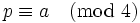.

## Proof

We need to check two congruence classes modulo: the congruence class ofand the congruence class of.

### The congruence class ofmoduloBy fact (2),is a quadratic residue moduloif and only if. In particular, a primecan divide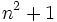for some natural numberif and only if.

Consider now the polynomial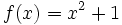. For any natural number, all prime divisors ofare congruent tomodulo. By fact (3), there are infinitely many pairwise relatively prime values of, so we get infinitely many primes that are congruent tomodulo.

### Congruence class ofmoduloBy fact (1),is a quadratic residue moduloif and only if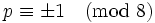. In particular, a primecan divide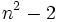for some natural numberif and only if.

Consider now the polynomial. For any natural number, all prime divisors ofare congruent to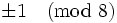. However,itself ismodulo, somust have at least one prime divisor that ismodulo. By fact (3), there are infinitely many pairwise relatively prime values of, yielding infinitely many distinct primes that aremodulo. In particular, all these primes aremodulo.